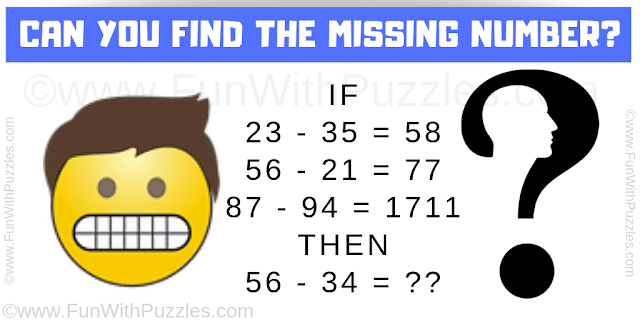## Thursday, February 21, 2019

This Maths Logic Puzzle will challenge your Brain. Solving this maths logical question will improve your logical reasoning and mathematical skills. In this puzzle, your challenge is to decipher the logical and mathematical pattern hidden in these given equations. Apply the same logic in the last equation to find the number which will replace the question mark.Can you solve this Maths Logic Puzzle?

Answer of this "Maths Logic Puzzle", can be viewed by clicking on the answer button.

1.1.